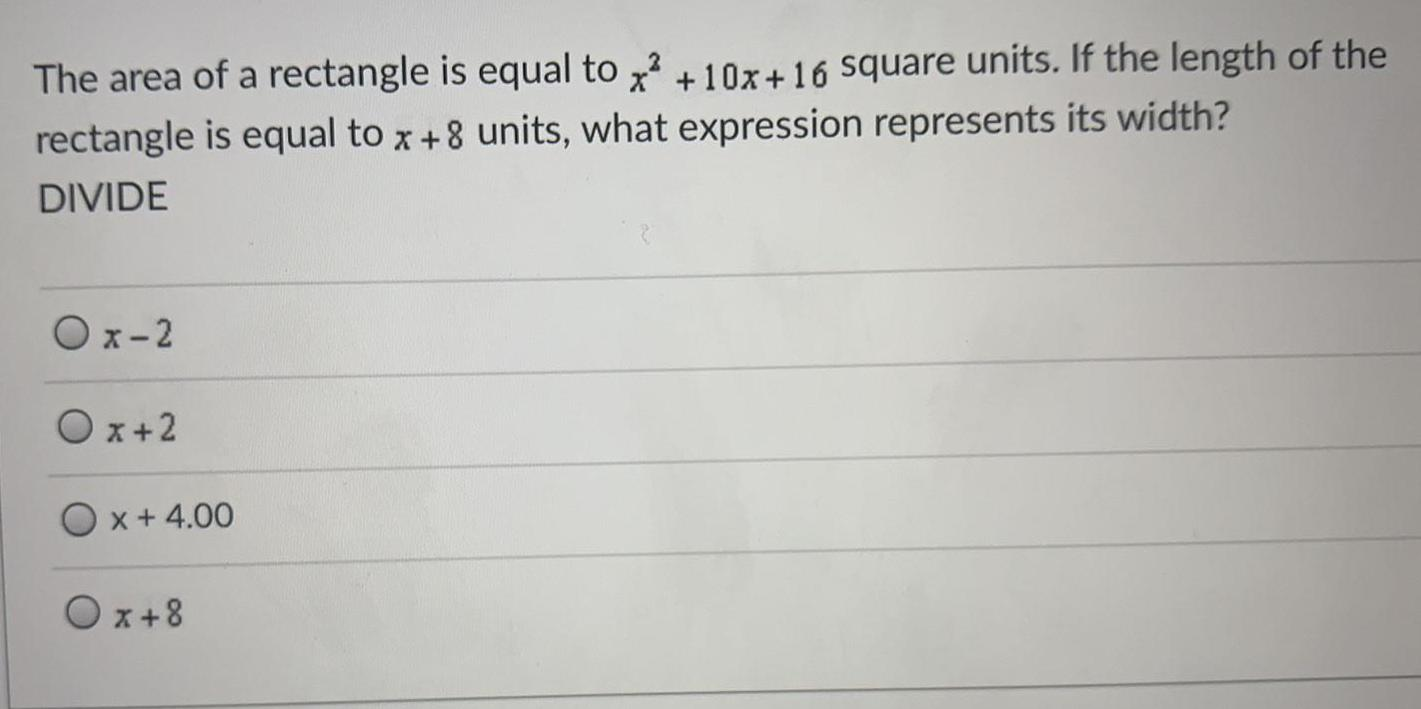Question:

# The area of a rectangle is equal to x² +10x+16 square unitsThe area of a rectangle is equal to x² +10x+16 square units. If the length of the rectangle is equal to x+8 units, what expression represents its width? DIVIDE x-2 x+2 x + 4.00 x+8